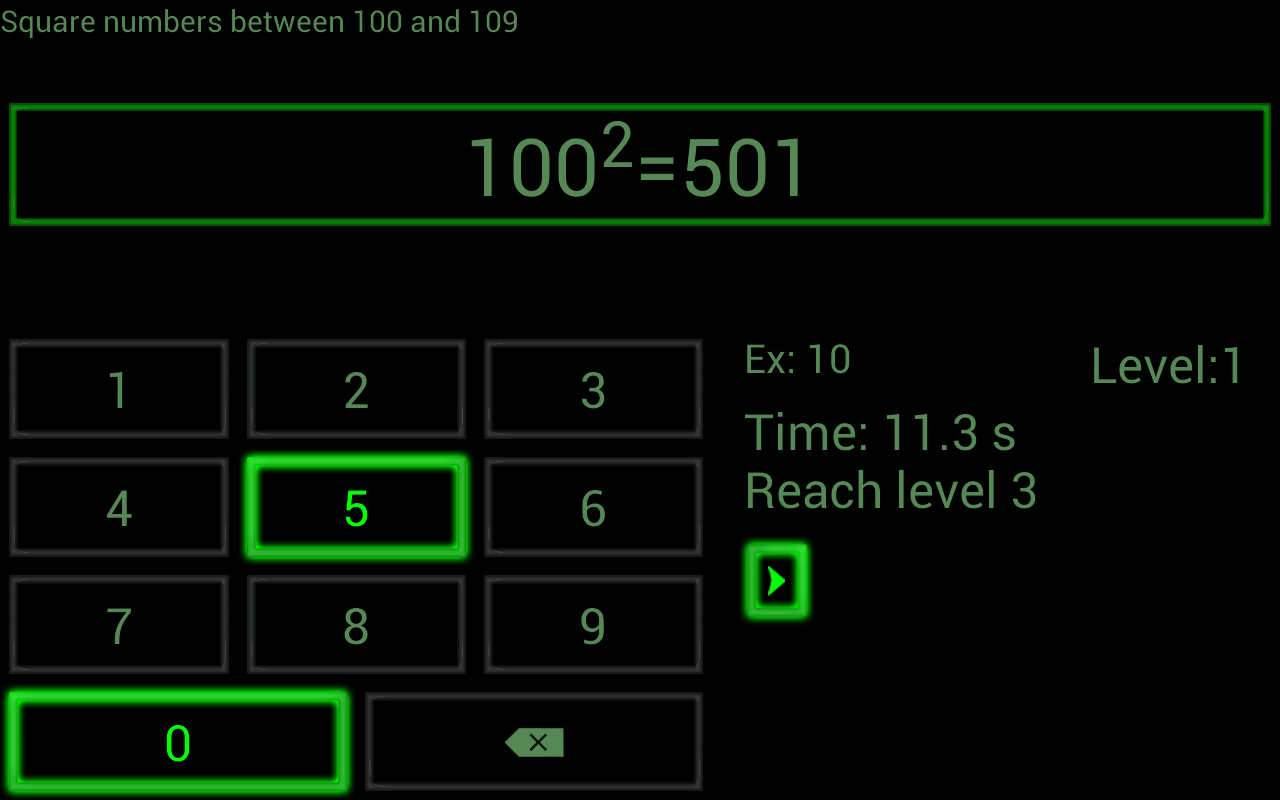# Material Detail## Math Tricks App for Android

'This program is dedicated to showing you a variety of interesting math tricks that will help you solve some basic math problems with great speed. You can play these tricks as instructed, with your friends and prove your talent to them. Fun Math Tricks: 1. Addition 2. Subtraction 3. Multiplication table 4. Division 5. Multiply two digit number by 11 6. Square numbers ending in 5 7. Multiply by 5 8. Multiply by 9 9. Multiply by 4 10. Dividing by 5 11. Subtracting from 1000 12. Tough multiplication 13. Power of two 14. Adding numbers close to hundreds 15. Subtracting numbers close to hundreds 16. Multiply numbers between 11 and 19 17. Square numbers between 11 and 99 18. Multiply two digit numbers having same tens digit and ones digits add up to ten 19. Square numbers between 50 and 59 20.... Show More
Rate

#### Quality

• Editor Reviews
• User Rating
• Learning Exercises
• Bookmark Collections
• Course ePortfolios
• Accessibility Info

#### Browse...

##### People who viewed this also viewed
••••##### Other materials like Math Tricks App for Android
••••